Physics
Fox

### Summary

• Every quantity we measure has units associated with it. For example, distance might be measured in units of centimetres, inches or feet.
•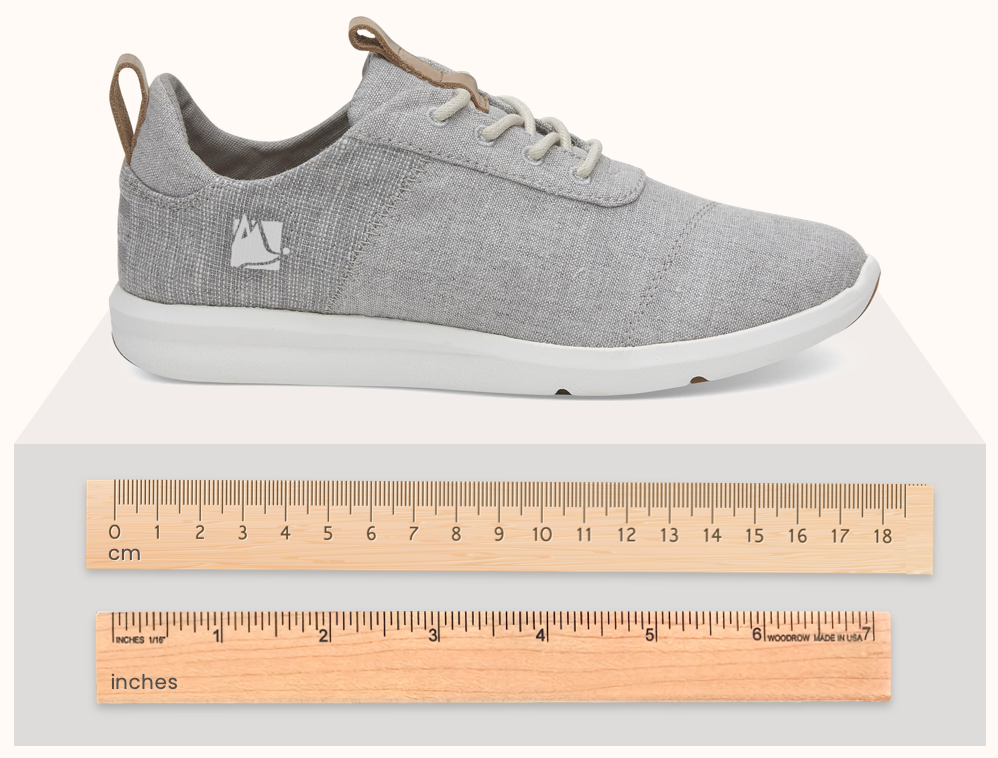This shoe has a length of 18cm, or about 7 inches.
(Since 1 foot = 12 inches, this shoe is smaller than a foot: making it very uncomfortable to wear 🙄)

• To keep things simple, in physics we use a standard set of scientific units for everything, known as SI units. For example, the SI unit of distance is metres.

• 'kilo' (k) in front of a unit makes it 1000 times bigger, and 'milli' (m) makes it 1000 times smaller.
•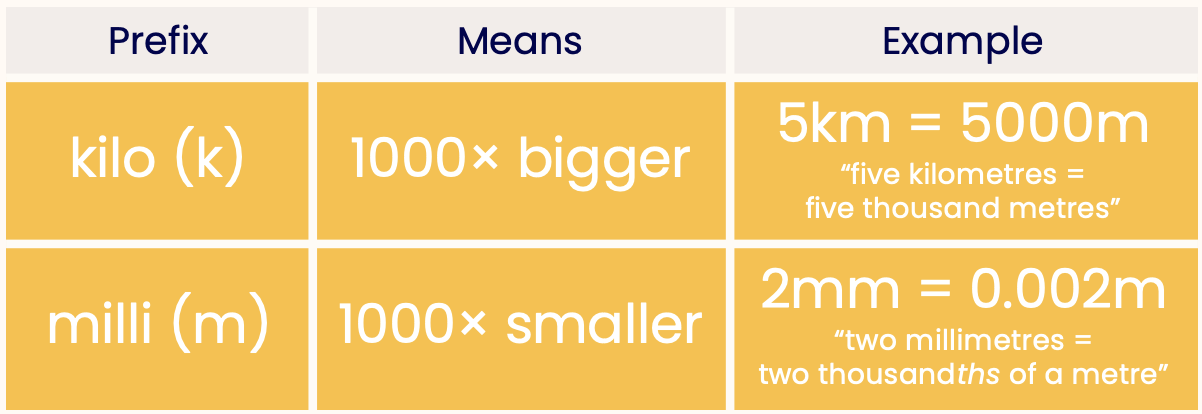• Any quantity you write down also needs a unit!
•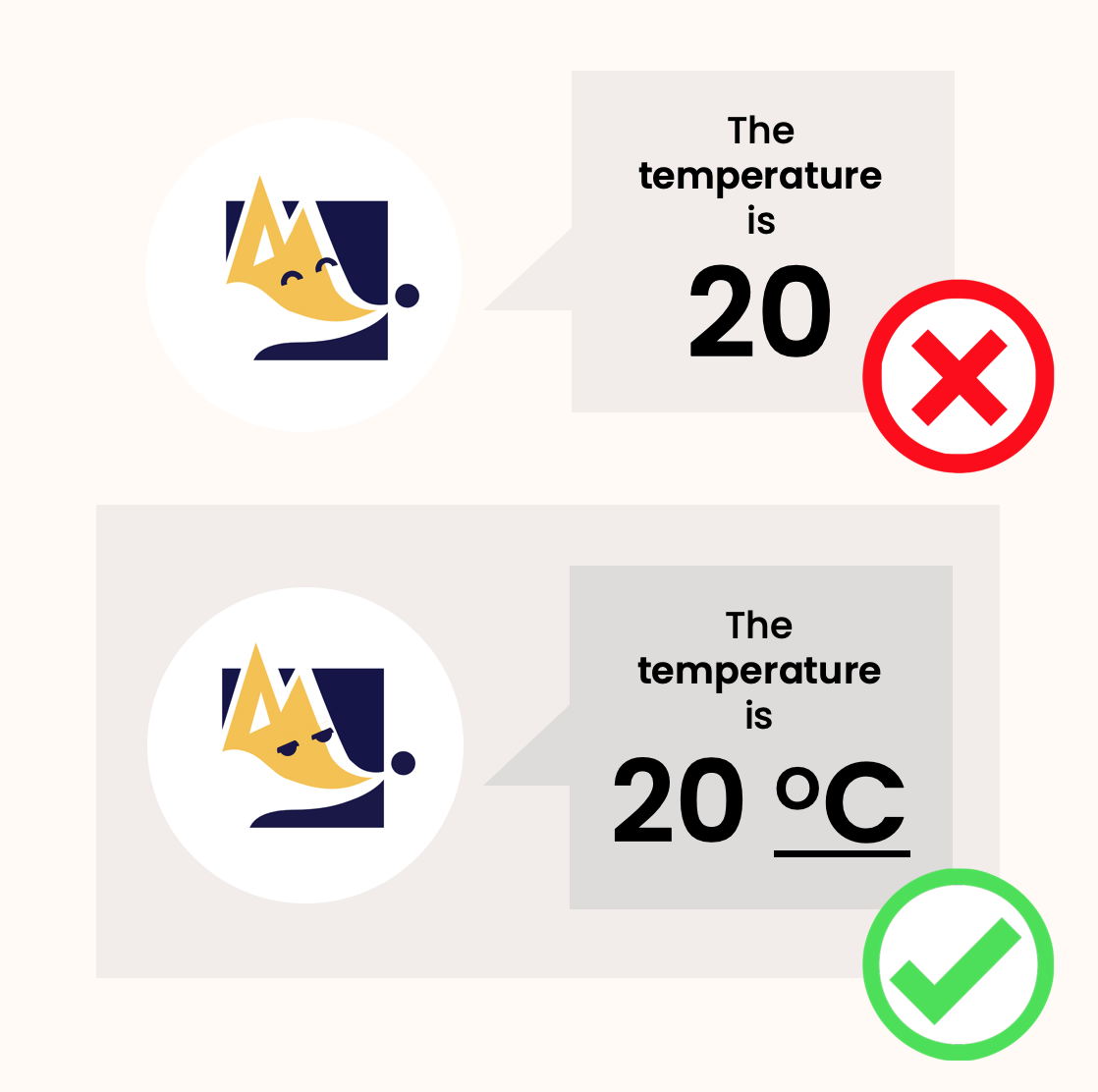TWOOO CHAAAAIIINZ

Hopefully you haven't found the quiz questions too challenging so far. Here's one you should find easy:

How old are you?
(this data isn't saved, don't worry)

All quantities have units associated with them.

For example, time can be measured in units of seconds, minutes, hours, days, etc.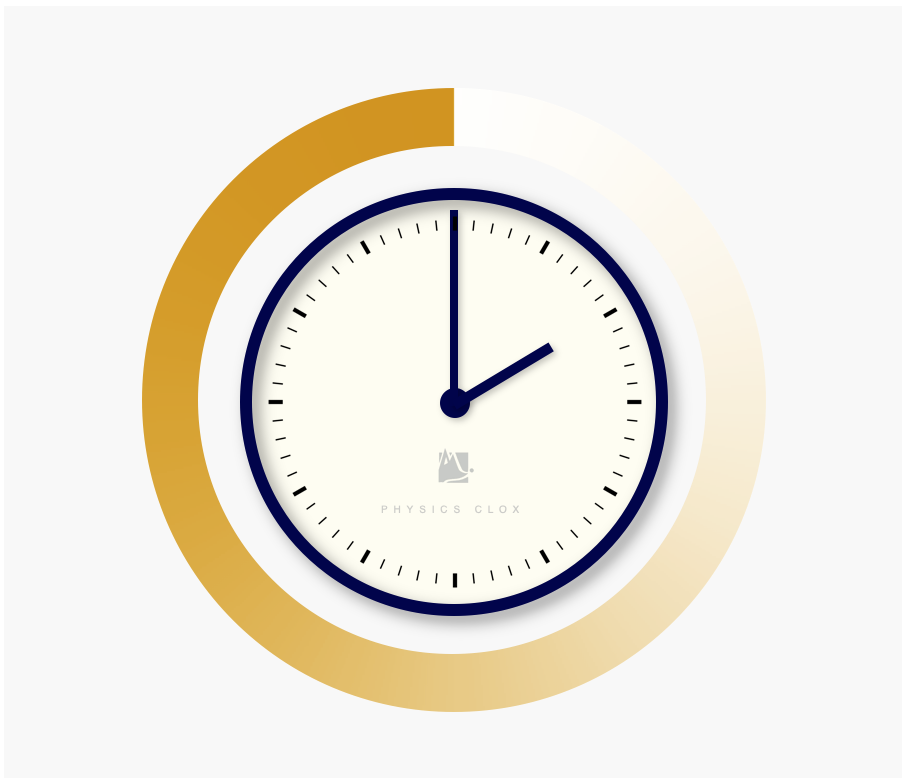1 hour = 60 minutes = 3600 seconds

Similarly, distance can be measured in units of centimetres (cm), inches, metres, feet, miles, kilometres (km).

As was painfully stated in the intro, any time you find a quantity, you need to have a unit with it. So saying a pencil is “5 long” doesn't mean anything, but saying it's “5 centimetres long” does mean something.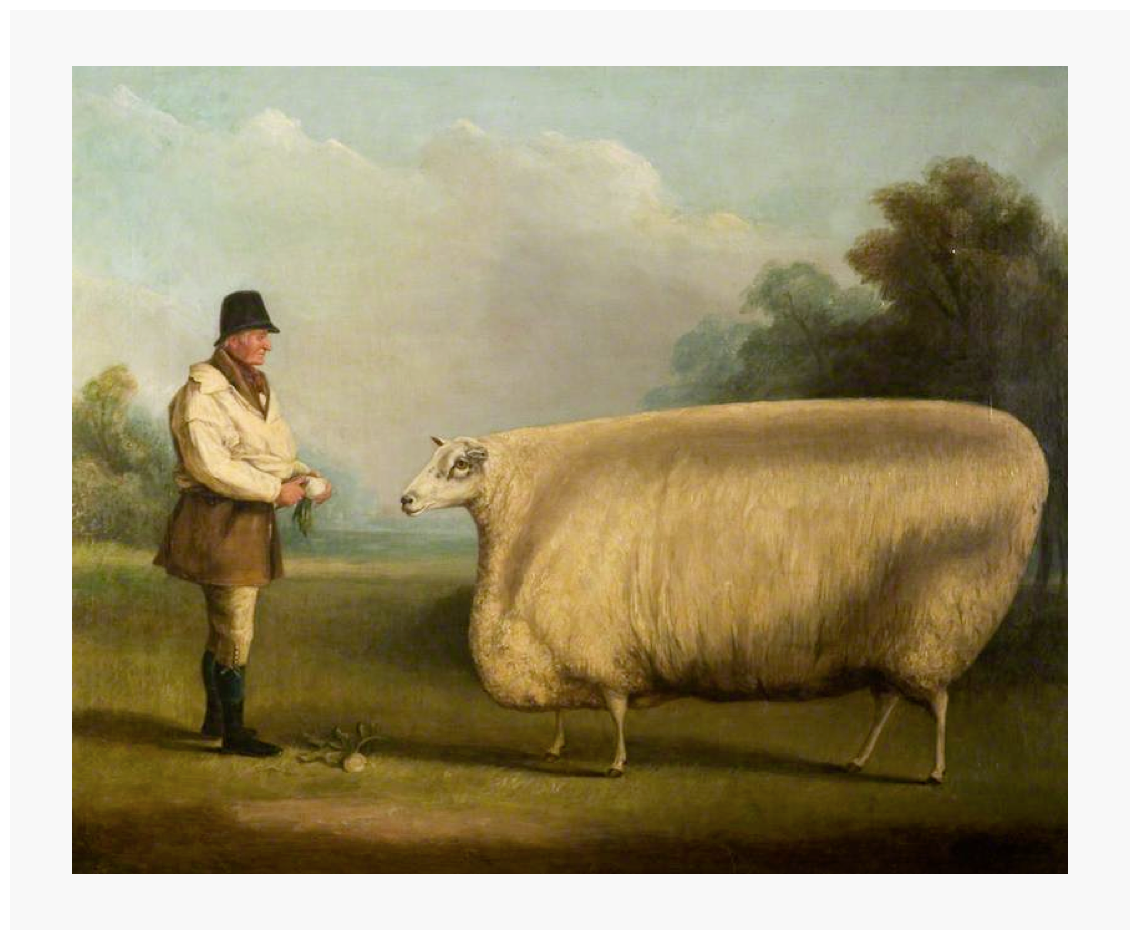An absolute unit.

Different units are more common in different places. For example, in the UK you would measure the width of mug in centimetres — but in the USA you would use inches. (That is, if you're the sort of strange person that goes around measuring mugs.)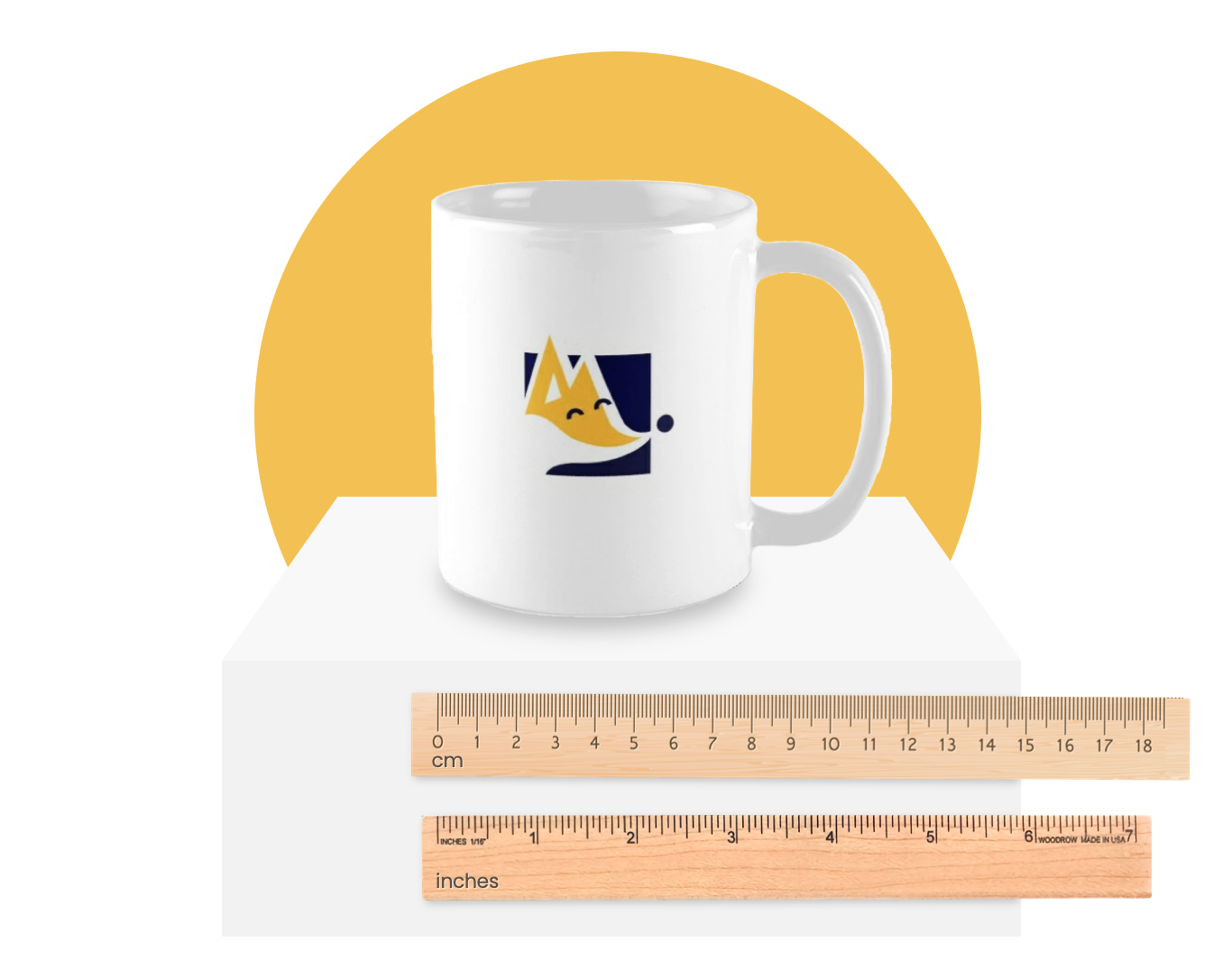This stylish mug has a width of 8cm, or about 3 inches.

It's a bit of a pain if everyone uses different units for measuring the same thing, because you have to keep converting other peoples' units to know what they mean.

In science, we get around this problem by agreeing to use the same set of units for everything.

This standard set of units are called scientific units, or SI units.

For example: the SI unit of distance is metres, and the SI unit of time is seconds.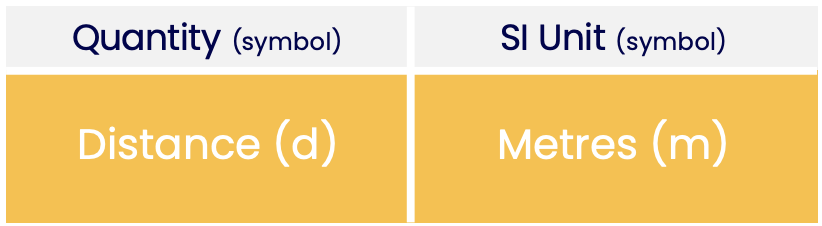Note that often we use a symbol to represent a unit (e.g. ‘m’ for metres). This is because we're lazy and can't be asked to write the whole word out every time.

In future, we will learn about more quantities and units. When we do, we'll use this box to show the name of the quantity and its SI unit.SI is short for Système International d’unités (French for International System of Units). It is also known as the metric system.

You might know that a kilometre is the same as 1000 metres. You might also know that a kilogram is 1000 grams.

1km = 1000m
1kg = 1000g

Similarly, a millimetre is a thousandth of a metre, and a millisecond is a thousandth of a second.

1mm = 11000 m = 0.001 m
1ms = 11000 s = 0.001 s

Maybe you can spot the pattern here — whenever kilo is used, it is a quantity 1000× bigger, and whenever milli is used, it is a quantity 1000× smaller.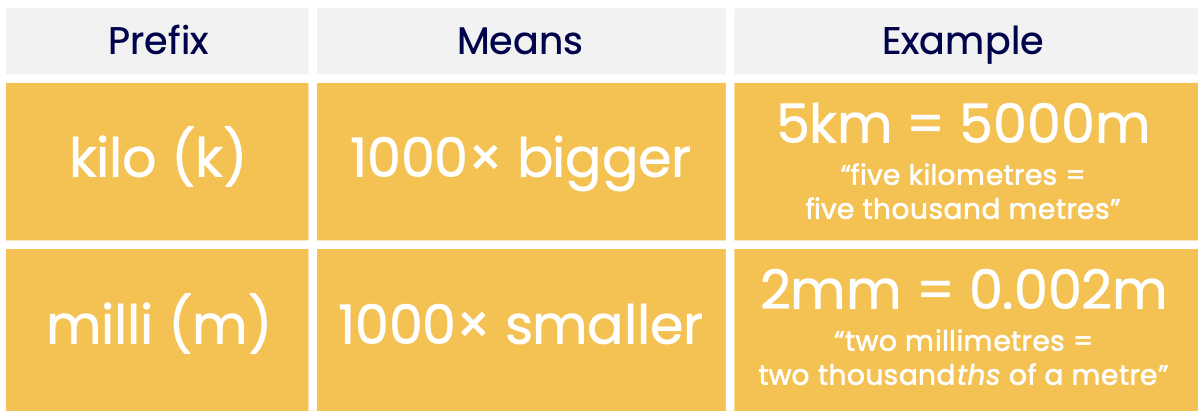For any scientific unit we use, we can always put 'kilo' or 'milli' in front of it when taking about large or small values.

For example, a grain of sand has a mass of about 15 mg (milligrams). A tiny amount!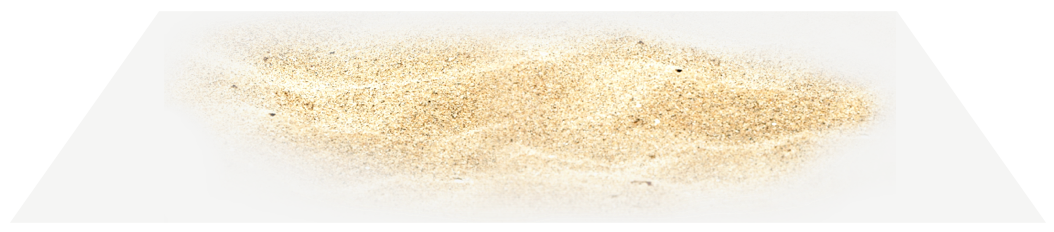Quiz

### Congratulations!

9 of 9 questions completed

+ ⭐️ collected.

• You can change your display name at any time.
• at least 1 letter
• at least 1 number
• at least 8 characters